Electron. J. Diff. Eqns., Vol. 2005(2005), No. 138, pp. 1-18.

### Nonlinear Kirchhoff-Carrier wave equation in a unit membrane with mixed homogeneous boundary conditions Nguyen Thanh Long

Abstract:
In this paper we consider the nonlinear wave equation problem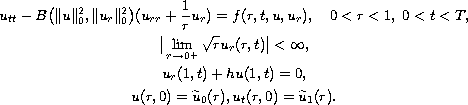To this problem, we associate a linear recursive scheme for which the existence of a local and unique weak solution is proved, in weighted Sobolev using standard compactness arguments. In the latter part, we give sufficient conditions for quadratic convergence to the solution of the original problem, for an autonomous right-hand side independent on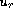and a coefficient function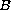of the form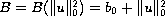with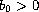.

Submitted August 3, 2004. Published December 1, 2005.
Math Subject Classifications: 35L70, 35Q72.
Key Words: Nonlinear wave equation; Galerkin method; quadratic convergence; weighted Sobolev spaces.

Show me the PDF file (308K), TEX file, and other files for this article.Nguyen Thanh Long Department of Mathematics and Computer Science University of Natural Science Vietnam National University HoChiMinh City 227 Nguyen Van Cu Str., Dist. 5 Hochiminh City, Vietnam email: longnt@hcmc.netnam.vn   longnt2@gmail.com